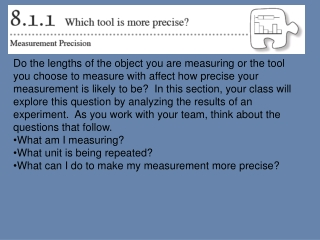DownloadDownload PresentationFor what values do class A's distribution and class B's distribution overlap?

# For what values do class A's distribution and class B's distribution overlap?

Télécharger la présentation## For what values do class A's distribution and class B's distribution overlap?

- - - - - - - - - - - - - - - - - - - - - - - - - - - E N D - - - - - - - - - - - - - - - - - - - - - - - - - - -
##### Presentation Transcript

1. Do the lengths of the object you are measuring or the tool you choose to measure with affect how precise your measurement is likely to be?  In this section, your class will explore this question by analyzing the results of an experiment.  As you work with your team, think about the questions that follow. • What am I measuring? • What unit is being repeated? • What can I do to make my measurement more precise?

2. Your Task: As directed by your teacher, measure the specified length in the classroom to the nearest inch twice, each time using a different tool (a 12-inch ruler and a single measuring tape, for example).  Although you will share tools with your team and work together to make sure the measures are recorded properly, each person in the team should measure the length twice, once with each tool. • 8-5. Creating visual representations of data makes data sets easier to compare. • Compare the center, shape, spread, and outliers for the two sets of data using the histograms and the box plots. Do the data sets seem to show the same value for the length of the classroom?

3. For what values do class A's distribution and class B's distribution overlap? Which measure could be used to compare the centers of the distributions? Which measure could be used to compare the spread of the two distributions? What is the mean of class A? What is the interquartile range of class B? Using the interquartile range of each class, compare the variabilities of the two classes.

4. 4. What is the mean of class A? 2.5 hamsters 5. What is the interquartile range of class B? Two pets 6. By how many pets is the center of class B's distribution greater than class A's center? The median number of pets in class B is two and a half pets more than the median number of pets in class A. 7. Using the interquartile range of each class, compare the variabilities of the two classes. The interquartile range (IQR) of class A is one pet, and the IQR of class B is 2 pets so the two classes have approximately the same variabilities with a difference of 1 7.SP.3: Worksheet Solutions The students in two seventh grade classes were asked, \How many hamsters have you seen on the side of the road in the past month?" The data was collected and a dot plot was created for each class. The two dot plots are shown in the graph below. (Class A's box plot is above class B's box plot.) 1. For what values do class A's distribution and class B's distribution overlap? The distribu- tions overlap from 2 to 5 hamsters. 2. Which measure should be used to compare the centers of the distributions? The means 3. Which measure should be used to compare the spread of the two distributions? The interquartile range

5. Practice Jason is comparing the height of players on his favorite soccer and basketball teams. He organized the information below. What is the range for each set of team?   What is the median for each team?  Does either team have an outlier? If so, list it. Which team is taller? Explain how you know. How do the means of each team compare?

6. Practice This table shows the marks (out of 50) obtained in some tests by two pupils: How do the ranges of each students data compare? What does this say about the students' work? How do the medians of each students data compare? What does this say about the students' work?